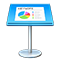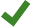# Calculate values using data in table cells

You can create formula or function cells that automatically perform calculations using the data in any cells you select. For example, you can compare values in two cells, calculate the sum or product of cells, and so on. The result of a formula or function appears in the cell where you entered it.

You can also use any of the 250 predefined mathematical functions included with Keynote to create formulas. There are functions for applications including statistics, finance, and engineering. Detailed information about each of these functions appears in the Function Browser, which appears when you indicate you’re adding a function to a cell (by typing an equal sign in the cell), and Formulas and Functions help online.

If you want to add an equation or expression to your presentation without performing a calculation, see Add an equation with MathType.

## Add, subtract, multiply, or divide values

You can create simple or complex arithmetic formulas to perform calculations on the values in your tables.

1. Click the cell where you want the result to appear, then type the equal sign (=).

The formula editor opens. Drag the left side of the formula editor to move it.

2. Select a cell to use as the first argument in your formula, or type a value (for example, a number such as 0 or 5.20).

3. Type an arithmetic operator (for example, +, -, *, or /), then select a cell to use as the next argument in your formula, or type a value.

By default, + is inserted between cell references. Drag the left side of the formula editor to move it.

5. Press Return or clickin the formula editor when you’re done.

If you click, you exit the formula editor without saving your changes.

## Compare values

You can use comparison operators to check whether the values in two cells are equal, or if one value is greater or less than the other. To do this, you must set up a statement within a cell, for example A1 > A2, meaning the value in cell A1 is greater than the value in cell A2. The result of the comparison operator is expressed as “true” or “false.”

1. Click the cell where you want the comparison result to appear, then type the equal sign (=).

The formula editor opens. Drag the left side of the formula editor to move it.

2. Select a cell whose value you want to compare, or type a value to compare.

3. Type a comparison operator (>, >=, =, <>, <, or <=), then select a cell whose value you want to compare, or type a value to compare.

4. Press Return or clickin the formula editor when you’re done.

If you click, you exit the formula editor without saving your changes.

## Insert functions in cells

You can use any of the 250 predefined mathematical functions included with Keynote in your presentation. The Function Browser includes examples showing how the functions work to help you choose one that suits your needs.

1. Click the cell where you want the result of the function to appear, then type the equal sign (=).

The formula editor opens, and the Function Browser appears on the right side of the window. Drag the left side of the formula editor to move it.

2. Type a function name (or terms associated with the function, such as “duration”) in the search field at the top of the Function Browser, or browse the available functions, then double-click the name of the function you want.

The function appears in the formula editor with all of the required and optional arguments for the function.

3. Select an argument in the function, then enter a value or select cells to include in the calculation by doing one of the following:

• Select a cell: Click the cell.

• Select a range of cells across multiple rows and columns: Drag across the range of cells you want to include.

• Add the values of a single row or column: Click the bar at the top of the column or to the right of the row—or select all of the cells in the column or row.

4. Press Return or clickin the formula editor when you’re done.

If you click, you exit the formula editor without saving your changes.

## Change the arguments of an existing function

You can edit an existing formula so that it refers to different cells.

1. Double-click the result cell with the formula you want to edit.

The formula editor opens, displaying the formula. Drag the left side of the formula editor to move it.

2. Do any of the following:

• Change the cell references: Select the existing cell addresses you want to change, then select the new cells.

• Remove cell references: Place the insertion point within the argument area for the function, select the unwanted cell references, then press Delete.

• Add more cell references: Place the insertion point within the argument area for the function, then select the new cells you want to add.

3. Press Return or clickin the formula editor when you’re done.

If you click, you exit the formula editor without saving your changes.

## Preserve row or column addresses in formulas

You can set row and column references in a formula to be absolute so that you can use the same formula somewhere else without changing the cell references.

If you don’t preserve the row or column references, then when you move the formula (by cutting and pasting it elsewhere or by adding new rows and columns to the table), the references are adjusted relative to the formula’s new location.

1. Double-click the result cell with the formula you want to edit.

The formula editor opens, displaying the functions. Drag the left side of the formula editor to move it.

2. Click the triangle on the token representing the cell range you want to preserve.

3. Select Preserve Row or Preserve Column for the start or end addresses of the selected range.

4. Press Return or clickin the formula editor when you’re done.

If you click, you exit the formula editor without saving your changes.